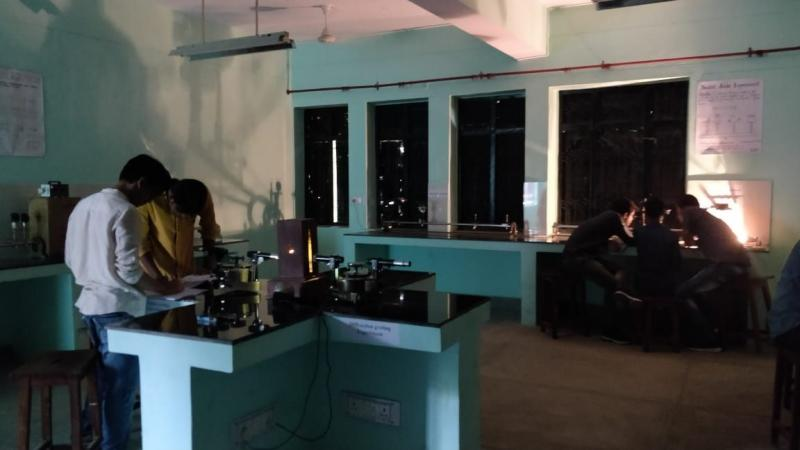# Physics LabIncharge
Prof. A. K. Katiyar

Room Number: ASD-207

Lab Faculty: Prof. A.K. Katiyar, Prof. O. P. Singh, Dr. Santosh K. Singh, Dr. Desh Deepak Tiwari

Lab Assistant: Mr. Gulam Jilani

Lab Instructor: Mr Nishakant Mishra

## Courses Covered

Physics (Practical)

Course Code: KAS-101/KAS-201

## List of Experiment

1. To determine the wavelength of monochromatic light (sodium light) by Newton’s Ring apparatus.
2. To determine the wavelength of a monochromatic light (sodium light) with the help of Fresnel’s Bi-prism.
3. To determine the wavelength of different spectral lines of mercury light using plane transmission grating.
4. To determine the specific rotation of cane sugar solution using biquartz polarimeter.
5. To determine the focal length of the combination of two lenses separated by a distance, and to verify the formula for the focal length of combination of lenses.
6. To determine resistance per unit length and resistance of the given wire using Carey Foster’s Bridge.
7. To determine electro-chemical equivalent (E.C.E.) of copper.
8. To verify Stefan’s law (E=σT4) by electric method.
9. To study the variation of magnetic field with distance along the axis of a current carrying coil and then to estimate the radius of the coil.
10. To determine the coefficient of viscosity of a given liquid by rotating cylinder method.

## Proposed Experiments to be installed

1.To determine the wavelength of He-Ne laser light using single slit diffraction.

2.To draw hysteresis (B-H curve) of a specimen in the form of a transformer and to determine its hysteresis loss.

3.To measure attenuation in an optical fiber.